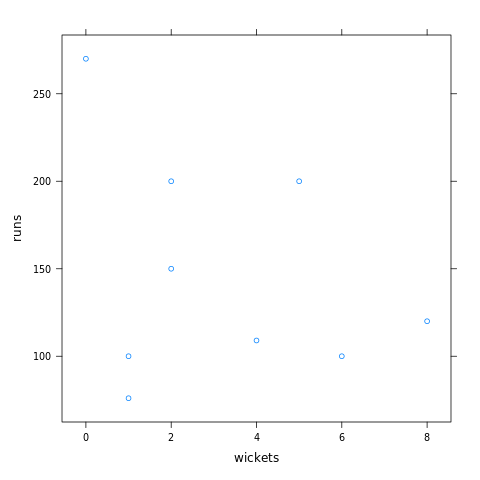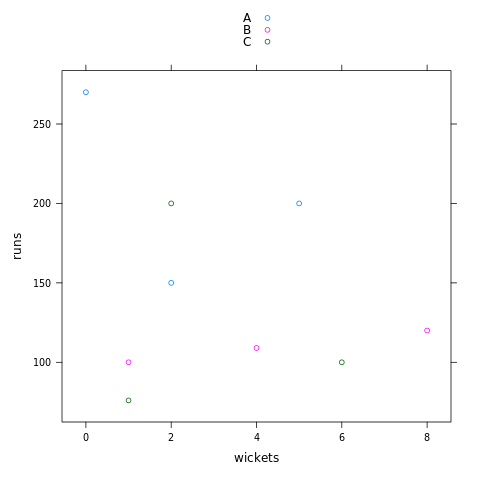# How to create a scatter plot using lattice package in R?

In this article, we will discuss how to create the scatter plots using lattice package in R programming language.

In R programming, the Lattice package is a data visualization library that consists of various functions to plot different kinds of plots. Using the lattice library we can able to plot various kinds of plots like scatter plot, Box plots, Histograms, 3D scatter plots, Dot plots, Strip plots, Density plots, etc. In order to use the functionalities of the lattice library need to import the library first.

To import the lattice library use the below statement-

library(lattice)

## Scatter plot using lattice package in R

In R, The Lattice library contains xyplot() method that is used to create a scatter plot. In order to use the xyplot() method, the lattice library needs to be imported first. The syntax of xyplot() method is given below-

Syntax: xyplot( col1~col2, data=dataframeName)

Let’s look into a couple of examples on how to plot the box plot using lattice library.

Example 1: In the below code we created a data frame “stats” and plotted a scatter plot between data in two columns using xyplot() method.

## R

 `# import lattice library ` `library``(lattice) ` ` `  `# create a data frame  ` `stats <- ``data.frame``(player=``c``(``'A'``, ``'B'``, ``'C'``, ``'A'``, ` `                             ``'B'``, ``'C'``, ``'A'``, ``'B'``,  ` `                             ``'C'``), ` `               ``runs=``c``(200, 100, 100, 150, 109,  ` `                      ``200, 270, 120, 76), ` `               ``wickets=``c``(5, 1, 6, 2, 4, 2, 0, 8, ` `                         ``1)) ` ` `  `print``(``"stats Dataframe"``) ` `stats ` ` `  `# groped scatter plot  ` `xyplot``(runs ~ wickets, data = stats)`

Output

```"stats Dataframe"
player runs wickets
1      A  200       5
2      B  100       1
3      C  100       6
4      A  150       2
5      B  109       4
6      C  200       2
7      A  270       0
8      B  120       8
9      C   76       1```Example 2: In this example, we plotted a grouped scatter plot for the above-created data frame using xyplot() method.

## R

 `# import lattice library ` `library``(lattice) ` ` `  `# create a data frame  ` `stats <- ``data.frame``(player=``c``(``'A'``, ``'B'``, ``'C'``, ``'A'``, ` `                             ``'B'``, ``'C'``, ``'A'``, ``'B'``,  ` `                             ``'C'``), ` `               ``runs=``c``(200, 100, 100, 150, 109, ` `                      ``200, 270, 120, 76), ` `               ``wickets=``c``(5, 1, 6, 2, 4,  ` `                         ``2, 0, 8, 1)) ` `                `  `# groped scatter plot  ` `xyplot``(runs ~ wickets, data = stats, group = player, auto.key = ``TRUE``)`

OutputWhether you're preparing for your first job interview or aiming to upskill in this ever-evolving tech landscape, GeeksforGeeks Courses are your key to success. We provide top-quality content at affordable prices, all geared towards accelerating your growth in a time-bound manner. Join the millions we've already empowered, and we're here to do the same for you. Don't miss out - check it out now!

Previous
Next